Courses

# Test: Circle- 4

## 30 Questions MCQ Test Mathematics For JEE | Test: Circle- 4

Description
This mock test of Test: Circle- 4 for JEE helps you for every JEE entrance exam. This contains 30 Multiple Choice Questions for JEE Test: Circle- 4 (mcq) to study with solutions a complete question bank. The solved questions answers in this Test: Circle- 4 quiz give you a good mix of easy questions and tough questions. JEE students definitely take this Test: Circle- 4 exercise for a better result in the exam. You can find other Test: Circle- 4 extra questions, long questions & short questions for JEE on EduRev as well by searching above.
QUESTION: 1

### The triangle PQR is inscribed in the circle x2 + y2 = 25. If Q and R have coordinates (3, 4) and (–4, 3) respectively, then ∠QPR may be equal to

Solution: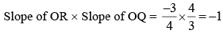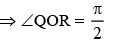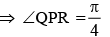(i.e. angle subtended at the centre of a circle is double the angle subtended in the alternate segment).
Hence (c) is the correct answer.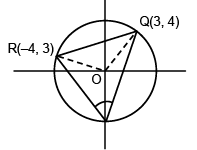QUESTION: 2

### If the circles x2 + y2 + 2ax + b = 0 and x2 + y2 + 2cx + b = 0 touch each other, then

Solution:

Since the circles touch each other, equation of the common tangent is S1 – S2 = 0 ⇒ x = 0.
Putting x = 0 in the equations of the circles, we get y2+ b = 0.
This equation should have equal roots ⇒ b = 0.
Hence (c) is the correct answer.

QUESTION: 3

### Two circles with radii ‘r1’ and ‘r2’, r1 > r2 > 2, touch each other externally. If ‘θ’ be  the  angle  between the  direct  common tangents,  then

Solution: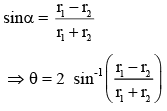Hence (B) is the correct answer.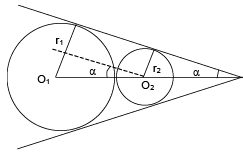QUESTION: 4

The common chord of x2 + y2 – 4x – 4y = 0 and x2 + y2 = 16 subtends at the origin an angle equal to

Solution:

The equation of the common chord of the circles x2+y2-4x-4y = 0 and x2+y2 = 16 is x + y = 4 which meets the circle x2+y2 = 16 at points A(4,0) and B(0,4). Obviously OA ⊥ OB. Hence the common chord AB makes a right angle at the centre of the circle x2+y2 = 16.
Hence (d) is the correct answer.

QUESTION: 5

A circle C and the circle x2 + y2 = 1 are orthogonal and have radical axis parallel to y-axis, then C can be

Solution:

(A) The  radical axis of the circle x2 + y2 = 1 and the circle given in is x = -2, which is | | to y-axis
(B) is y = 2, which is | | to x-axis
(C) is x = 0, which is y-axis
The radical axis with circle in (A) | | to y-axis and the given circle intersect circle given in A orthogonally.

QUESTION: 6

The tangents drawn from the origin to the circle x2 + y2 + 2gx + 2fy + f2 = 0 are perpendicular if

Solution:

Radius of the given circle = |g|. For perpendicular tangents origin should lie on the director circle, i.e. distance of origin from the centre of the given circle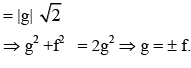QUESTION: 7

If one of the diameters of the circle x2 + y2 - 2x - 6y + 6 = 0 is a chord to the circle with centre (2, 1), then the radius of the circle is

Solution:

Centre is (1, 3) and radius = 2
If r = radius of second circle then r2 = 22 + (3 - 1)2 + (2 - 1)2
⇒ r = 3.
Hence (C) is the correct answer.

QUESTION: 8

Let A0 A1A2A3 A4A5 be a regular hexagon inscribed in a circle of unit radius. Then the product of the lengths of the line segments A0A1, A0 A2 and A0 A4 is

Solution: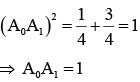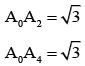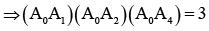Hence (C) is the correct answer.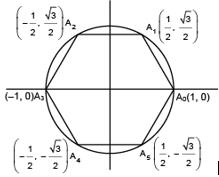QUESTION: 9

If the point (k + 1, k) lies inside the region bound by the curve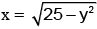and the y-axis, then k belong to the interval

Solution:

Since (k + 1, k) lies inside the region bounded by x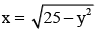⇒ (k + 1)2 + k2 - 25 < 0
and k + 1 > 0
⇒ 2k2 + 2k - 24 < 0 and k > -1
⇒ -4 < k < 3 and k > -1
⇒ -1 < k < 3
Hence (A) is the correct answer.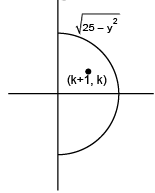QUESTION: 10

A line meets the coordinate axes in A and B, and a circle is circumscribing triangle AOB where O is the origin. If m, n are the distances of the tangents to this circle at the origin from the points A and B respectively, then the diameter of the circle is

Solution:

Since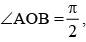AB is the diameter of the circle.
From figure,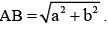Also equation of the circle is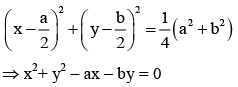equation of tangent to this circle at (0, 0) is ax + by = 0 ……(1)
∴ m = length of perpendicular from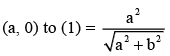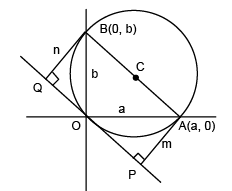and n = length of perpendicular from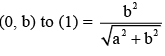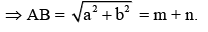Hence (D) is the correct answer.

QUESTION: 11

The locus of the centre of a circle which passes through the origin and cuts off a length 2b from the line x = c, is

Solution:

Let the centre be C (h, k). Since the circle passes through the origin,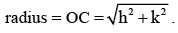Let x = c meets the circle in A and B.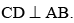From figure, CB2 = CD2 + BD2
⇒ OC2 = CD2 + b2  (∴CB=OC and BD=b)
⇒ h2 + k2 = (h - c)2 + b2
⇒ k2 + 2ch - c2 - b2 = 0
⇒ locus is y2 + 2cx = b2 + c2.
Hence (C) is the correct answer.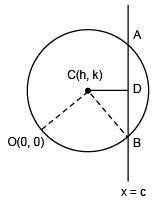QUESTION: 12

If the curves ax2 + 4xy + 2y2 + x + y + 5 = 0 and ax2+ 6xy + 5y2+ 2 x + 3y + 8 = 0 intersect at four concyclic points then the value of a is

Solution:

Any second degree curve passing through the intersection of the given curves is ax2 + 4xy + 2y2 + x + y + 5 + λ (ax2 + 6xy + 5y2 +2x + 3y + 8) = 0
If it is a circle, then coefficient of x2 = coefficient of y2 and  coefficient of xy = 0 a(1+ λ) = 2 + 5λ and   4 + 6λ = 0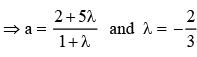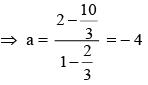Hence (B) is the correct answer.

QUESTION: 13

Equation of chord AB of circle x2 + y2 = 2 passing through P(2, 2) such that PB/PA = 3, is given by

Solution:

Any line passing through (2, 2)  will be  of  the  form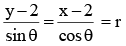When this line  cuts  the  circle  x2+y2=2 , (rcosθ+2)2 +(rsinθ+2)2 = 2
⇒ r2 + 4(sinθ + cosθ)r+6 = 0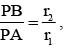now if r1 = α, r2 = 3α then 4α
=  - 4(sinθ + cosθ), 3α2 = 6 ⇒ sin2θ = 1⇒ θ = π/4 .
So required chord will be y – 2  = 1 (x –2) ⇒ y = x.
Alternative solution
PA.PN = PT2 = 22 + 22 - 2 = 6
PB/PA = 3
From (1) and (2), we have PA = √2, PB = 3 √​2
⇒ AB = 2 √​2
Now diameter of the circle is 2 √​2(as radius is √​2)
Hence line passes through the centre ⇒ y = x .
Hence (B) is the correct answer.

QUESTION: 14

If P(2, 8) is an interior point of a circle x2 + y2 –2x + 4y – p = 0 which neither touches nor intersects the axes, then set for p is

Solution:

For internal point p(2, 8),  4 + 64 – 4 + 32 – p < 0 ⇒ p > 96
and x intercept =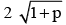therefore 1 + p < 0
⇒ p < -1 and  y intercept =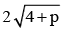⇒ p < -4
Hence (D) is the correct answer.

QUESTION: 15

Radii of the smallest and the largest circle passing through a point lying on the sides of a rectangle with vertices (± 2, ± 1) and touching the circle x2 + y2 = 9, are r1 and r2 respectively. Let d = |r1 – r2| then minimum value of d is

Solution: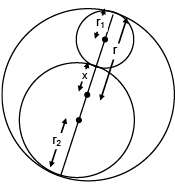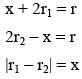⇒ |r2 - r2| is minimum when x is minimum.
|r2 - r2| = 1

QUESTION: 16

If the point (2cosθ, 2sinθ) does not lie in the angle between the lines x + y = 2  and x -y = 2 in which the origin lies, then number of solutions of the  equation √2 + cosθ + sinθ = 0  is

Solution:

The point (2 cosθ, 2 sinθ) lies on the circle x2 + y2 = 4. From the figure, it  is  obvious that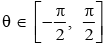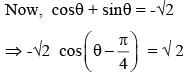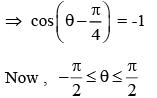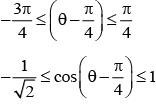Hence no solution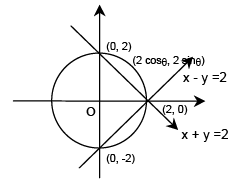QUESTION: 17

The radical axis of the two distinct circles x2 + y2 + 2gx + 2fy + c = 0 and 2x2 + 2y2 + 4x + y + 2c = 0 touches the circle x2 + y2 - 4x - 4y + 4 = 0. Then the centre of the circle x2 + y2 + 2gx + 2fy + c = 0 can be

Solution:

The radical axis of given circles is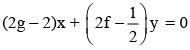This line is tangent to the circle x2 + y2 – 4x – 4y + 4 = 0
⇒ either g = 1 or f = 1/4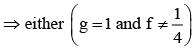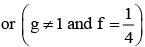QUESTION: 18

Equation of a circle that cuts the circle x2 + y2 + 2gx + 2fy + c = 0, lines x = – g and y = –f orthogonally, is;

Solution:

Clearly (-g, -f) will be the centre of required circle,
Let the circle be (x + g)2 + (y + f)2 = d
⇒ x2 + y2 + 2gx + 2fy + g2 + f2 –d = 0
It has to cut the given circle orthogonally,
⇒ 2g2 + 2f2 = c + g2 + f2 –d
⇒ d = c –g2 –f2

QUESTION: 19

The range of values of α for which the line 2y = gx + a is a normal to the circle x2 + y2 + 2gx +2g y - 2 = 0 for all values of g is

Solution:

The line 2y = gx + β should pass through (–g, –g) so,
–2g = –g2 + α ⇒ α = g2 – 2g = (g – 1)2 – 1 > –1.

QUESTION: 20

The point of intersection of the tangents of the circle x2 + y2 = 10, drawn at end points of the chord x + y = 2 is

Solution:

Let (h, k) be point of intersection of the tangents Then equation of chord of contact is xh + yk = 10
Compare this with x +y = 2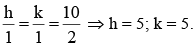QUESTION: 21

The line x + y = 5 intersects the circle x2 +y2 - 6x - 8y + 21 = 0 at points A and B, then the locus of the point C such that AC is perpendicular to BC is

Solution:

Since AB is fixed and AC is perpendicular to BC. So, locus of C is a circle whose diameter is AB. So, family of circles passing through AB is
x2 + y2 - 6x - 8y + 21+λ(x + y -5) = 0
⇒x2 + y2 - (6 - λ)x - (8 - λ)y + 21- 5λ = 0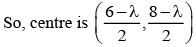Since AB is diameter so centre must lie on AB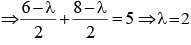∴ Locus is x2 + y2 - 4x - 6y + 11 = 0.

QUESTION: 22

If a circle of radius 3 units is touching the lines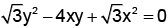in the first quadrant then length of chord of contact to this circle is

Solution:

Given equation of lines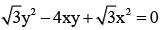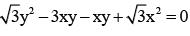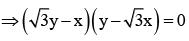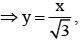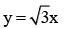∠APO = 75°
Length of chord of contact AB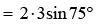= 6(sin45°cos30° + sin30°cos45°)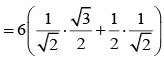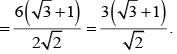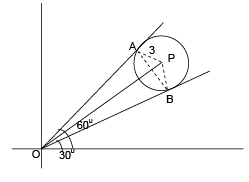QUESTION: 23

(x – 1)(y – 2) = 5 and (x – 1)2 + (y + 2)2 = r2 intersect at four points A, B, C, D and if centroid of ΔABC lies on line y = 3x - 4, then locus of D is

Solution:

If (xi, yi) is the point of intersection of given curves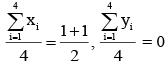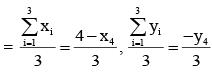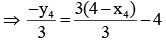⇒ y4 = 3x4 ⇒ locus of D is y = 3x.

QUESTION: 24

Tangents PA and PB are drawn to circle (x - 5)2 + (y - 7)2 = 1 from point P lying on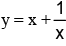Locus of circumcentre of triangle PAB is

Solution: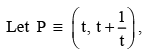now if centre of circle is(5, 7) ≡ C say then PACB is a cyclic quadrilateral, so circumcentre of triangle PAB is midpoint of PC i.e.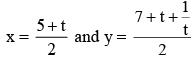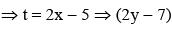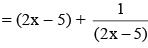⇒ 4x2 - 4xy - 6xy + 10y - 10 = 0

QUESTION: 25

The point on the straight line x + y = 2, which is nearest to the circle x2 + y2 –10x + 2y + 22 = 0

Solution:

Shortest distance between 2 curves occurs along the common normal. Let required point be (t, 2 -t). Normal at this will be y –(2 –t) = (x –t), it should passes through centre of circle so –1 –2 + t = 5 –t ⇒ t = 4, so point can be (4, -2).

QUESTION: 26

Formula used to measure circumference of circle is

Solution:
QUESTION: 27

In formula 2πr, 'r' is considered as

Solution:
QUESTION: 28

If circumference of circle is 64π then area of circle (in terms of π) is

Solution:
QUESTION: 29

Formula used to measure area of circle is

Solution:
QUESTION: 30

If circumference of circle is 82π then value of 'r' is

Solution: